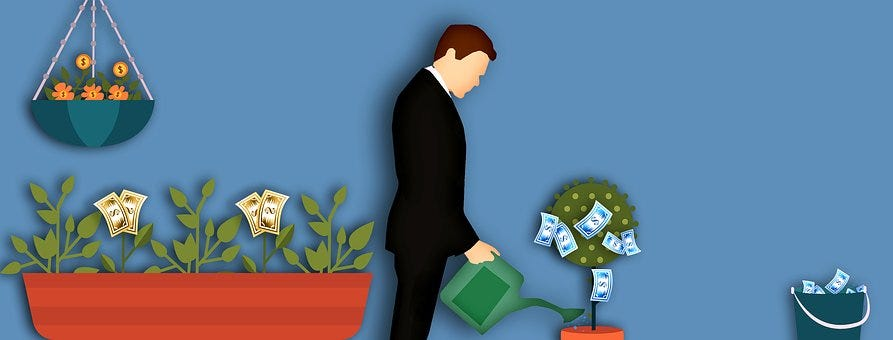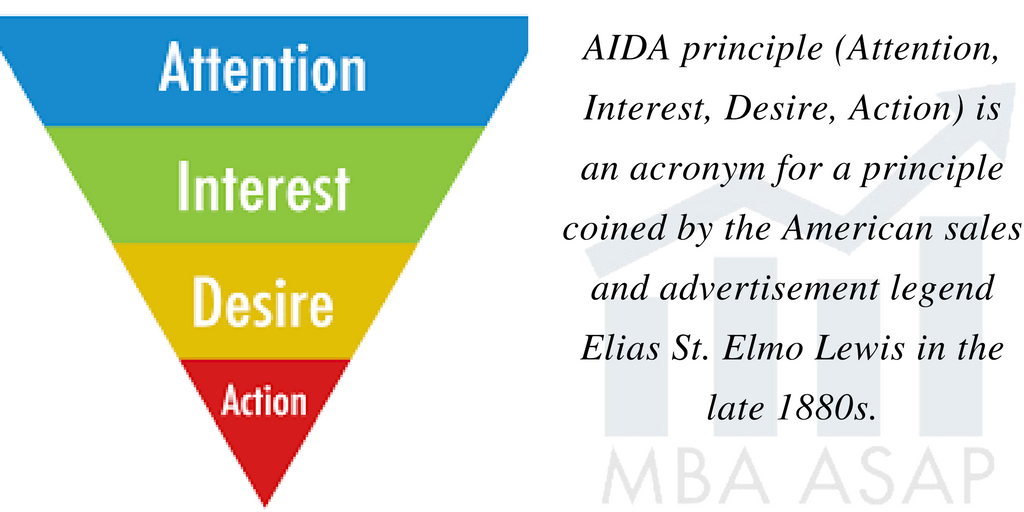# Cost-Volume-Profit Analysis: Figuring out how you get to profitabilityJohn Cousins
February 7, 2023Cost–volume–profit (CVP) is an analytic tool based on cost accounting measures. CVP analysis is a framework for figuring out how you get to profitability. Achieving consistent profitability is how you create a sustainable business.

CVP is very useful for making informed decisions and analysis.

CVP analysis expands on break-even analysis. An important transition point in CVP analysis is the break-even. This is where total revenues equal total costs. At break-even an enterprise has no profit or loss and costs are covered.

CVP is based on the same assumptions as break-even analysis:

· Costs and revenues behave linearly.

· Costs are either fixed or variable.

· The amount of activity is the only factor affecting costs.

· All the units produced are sold. There is no inventory build up.

· The product mix remains constant.

The CVP elements are:

· Activity Level

· Unit prices

· Unit Variable Cost

· Total fixed costsNow that I’m done painting I should go figure out how many units I need to sell to be profitable.

Economics is considered a science. Science seeks to gain insights by using assumptions to reduce complexity. Managerial economics reduces the complexity of situations to gain better understanding and insight. This insight is helpful for informed decision making and future planning.

eepurl.com

CVP assumptions create a simplified linear model of how costs and profits interact. This interaction plots levels of volume sold at a consistent unit price. Increases in volume effect total revenues and costs in a linear fashion. This is because we assume constant costs and prices.

CVP identifies the contribution of revenue to cover fixed costs. This contribution margin is what is left after variable costs are covered. The nature of the contribution margin is the main insight of CVP. If the unit price is greater than the unit variable cost, then each successive unit sold will chip away at fixed costs. The break-even point is a special case of CVP where revenue covers total fixed and variable costs.

The following are the formulas for deriving CVP:

Total Costs = Total Fixed Costs + (unit variable costs X number of units)

Total Revenues = Sales Price X number of units

Now we need a little algebra. Don’t be intimidated. We are just putting the elements of the above formulas into abbreviations:

TC = FC + V x U

TR = P x U

Where:

· TC = Total costs

· FC = Total fixed costs

· V = Unit variable cost

· U = Number of units

· TR = Total revenue or Sales

· P = Sales price per unit

We calculate profit as TR-TC. It is a profit if this is a positive number and a loss if it is negative.

Next we unbundle the components of Costs and Sales. This will provide further insight into operations. We will do this by deriving a formula for the contribution margin. This will take a bit more algebraic manipulation. Hang with me and don’t let the algebra intimidate you. It will follow the narrative description above about what the Contribution Margin means.

First, we separate total costs into the fixed and variable costs components:

TC = FC + V x U

We can now unbundle the components of sales as contribution plus variable costs. Contribution is what’s left after deducting variable costs from sales. Before achieving break-even, contribution goes towards offsetting fixed costs. After breakeven, contribution is the amount of a unit sale going towards profit.

TR = C x U + V x U

We can think of profit/loss (PL) as the contribution margin from the units sold minus the total fixed costs:

PL = C x U — TFC

CVP analysis is a framework for figuring out how you get to profitability. It describes how many units at what price you need to sell to cover all your costs. In practice, the assumptions can limit its accuracy. It is a way to get a handle on the dynamics of how your cost structure affects your goal of turning a profit. And turning a profit is how you remain a sustainable business.John Cousins
Author, Entrepreneur, & Teacher

### Receive my 7 day email course

Take your finance skills to the next level with my 7-day corporate finance email course. You'll learn all the essential topics from financial analysis to risk management in a fun, engaging format. Each day, you'll receive an email with practical examples, exercises and resources. Perfect for aspiring finance pros or anyone looking to expand their knowledge. Get ready to transform your finance game!

By clicking Sign Up you're confirming that you agree with our Terms and Conditions.
Oops! Something went wrong while submitting the form.

## If you like this article. Here are some more articles I think you might like.Entrepreneurship

### AIDA Marketing Framework: Attention, Interest, Desire, ActionJohn Cousins
November 14, 2023Personal Growth

### A Pause for the Cause: Don’t get mad, don’t get even, Get What You Want.

Pause and breath. You can’t unring a bell. Don’t say things when you are hot that you will later regret.John Cousins
November 14, 2023